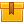# Electrical Schematics (A8004)

## Prerequisites

This lesson is designed so that no prior knowledge is required.

## Description

This lesson shows and explains how to read and interpret the symbols on an electrical schematic the function of the input, logic, and output elements of a control circuit. This lesson identifies devices that are typically used as these elements, and presents their schematic symbols. This lesson also describes the steps for interpreting the relationships among the input, logic, and output components of an electrical schematic.

## Objectives

Explain the function of the input, logic, and output element of a control circuit. Identify the symbol for various manually operated input devices, and identify symbols for various process? actuated input devices and state how they are used. Identify the symbol for a relay and the associated contacts. Identify various logic symbols, the symbol for a motor starter, and various output symbols and state how they are used. Describe the layout of a typical electrical schematic and describe various conventions for labeling schematics. Interpret an electrical schematic and know how to state the functions of its devices.

## ContentThis lesson shows and explains how to read and interpret the symbols on
an electrical schematic the function of the input, logic, and output
elements of a control circuit. This lesson identifies devices that are
typically used as these elements, and presents their schematic symbols.
This lesson also describes the steps for interpreting the relationships
among the input, logic, and output components of an electrical
schematic.

•Electrical Schematics

0
0 Reviews